Checkout JEE MAINS 2022 Question Paper Analysis : Checkout JEE MAINS 2022 Question Paper Analysis :

# Torque On Current Loop

Let us consider a rectangular loop such that it carries a current of magnitude I. If we place this loop in a magnetic field, it experiences a torque but no net force, quite similar to what an electric dipole experiences in a uniform electric field.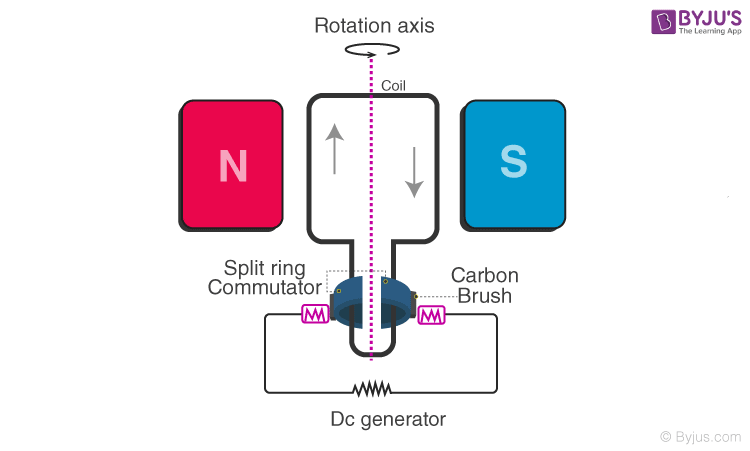## Torque On Current Loop

Let us now consider the case when the magnetic field B is in the plane with the rectangular loop. No force is exerted by the field on the arms of the loop that is parallel to the magnets, but the arms perpendicular to the magnets experience a force given by F1,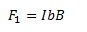This force is directed into the plane.

Similarly, we can write the expression for a force F2 which is exerted on the arm CD,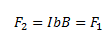We see that the net force on the loop is zero and the torque on the loop is given by,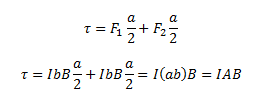Where ab is the area of the rectangle. Here, the torque tends to rotate the loop in the anti-clockwise direction.

Let us consider the case when the plane of the loop is not along the magnetic field. Let the angle between the field and the normal to the coil be given by θ. We can see that the forces on the arms BC and DA will always act opposite to each other and will be equal in magnitude. Since these forces are the equal opposite and collinear at all points, they cancel out each other’s effect and this results in zero-force or torque. The forces on the arms AB and CD are given by F1 and F2. These forces are equal in magnitude and opposite in direction and can be given by,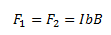These forces are not collinear and thus act as a couple exerting a torque on the coil. The magnitude of the torque can be given by,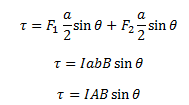### Torque On Current Loop – The Magnetic moment

The magnetic moment of a current loop can be defined as the product of the current flowing in the loop and the area of the rectangular loop. Mathematically,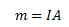Here, A is a vector quantity with the magnitude equal to the area of the rectangular loop and the direction is given by the right-hand thumb rule. In the equation written earlier, we can see that the torque exerted on a current-carrying coil placed in a magnetic field can be given by the vector product of the magnetic moment and the magnetic field.## Frequently Asked Questions – FAQs

### What is meant by torque?

Torque is the rotational analogue of linear force. Depending on the topic, it is also termed the moment of force, the moment, the turning effect, or the rotational force.

### What is current?

Current can be simply defined as the flow of charge through a space or a conductor.

### What is a magnetic field?

A magnetic field is a field that shows the magnetic influence on magnetic materials, moving charges, and electric currents.

### What is an electric dipole?

An electric dipole is a couple of opposite charges -q and q divided by a length ‘d’.

### Explain the magnetic moment of a current loop.

The magnetic moment of a current loop can be defined as the product of the current flowing in the loop and the area of the rectangular loop. Mathematically,
m=IA

Test Your Knowledge On Torque Current Loop!